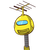# can 20men WUNng a nous pui cu4.175 men can dig a canal 3150 m long in 36 days. How many men are required to dig a January 6, 2022 by Eden

can 20men WUNng a nous pui cu
4.
175 men can dig a canal 3150 m long in 36 days. How many men are required to dig a
canal 3900 m. long in 24 days?
of a book.​

### 1 thought on “can 20men WUNng a nous pui cu<br />4.<br />175 men can dig a canal 3150 m long in 36 days. How many men are required to dig a<br /”

1.Answer:In 36 days 175 men dig 3150 m long canal.

one man in one day digs 3150/(36×175) m long canal.

one man in 24 days can dig (3150×24)/(36×175) m long canal.

to dig 3900 m long canal in 24 days ,no of men is to be provide =3900÷{(3150×24)/(36×175)}

=(3900×36×175)/(3150×24)

=(78×50×12×3×175)/(63×50×12×2)

=(78×3×175)/(63×2)

=(39×175)/(21)

=(13×175)/7

=13×25

=325

325 men are required

Step-by-step explanation: